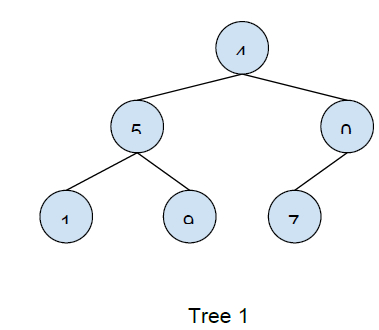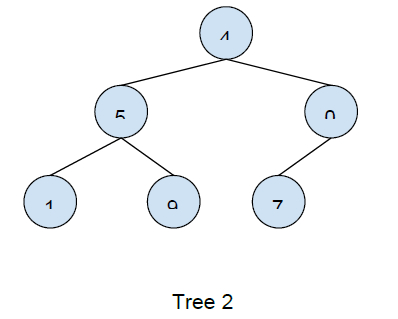# Write Code to Determine if Two Trees are Identical in C++

In this problem, we are given two trees. Our task is to write a code to check whether the two trees are identical or not.

Two trees are said to be identical if elements of the arrays have the same value and orientation.

ExampleAs both of the trees have the same values and position of elements both trees are identical.

To check if two trees are identical, we will go from node node to each node of the and check for their equality step by step and if at any point to nodes are not equal return -1, denoting the tree are not identical and if the whole tree is traversed or both trees are empty return 1, indicating the trees are identical.

Program to illustrate the working of the above solution,

## Example

Live Demo

#include <iostream>
using namespace std;
class node{
public:
int data;
node* left;
node* right;
};
node* insertNode(int data){
node* Node = new node();
Node->data = data;
Node->left = NULL;
Node->right = NULL;
return(Node);
}
int isIdentricalTrees(node* tree1, node* tree2){
if (tree1 == NULL &amp;&amp; tree2 == NULL)
return 1;
if (tree1 != NULL &amp;&amp; tree2 != NULL){
return( tree1->data == tree2->data &amp;&amp; isIdentricalTrees(tree1->left,
tree2->left) &amp;&amp; isIdentricalTrees(tree1->right, tree2->right) );
}
return 0;
}
int main(){
node *root1 = insertNode(4);
node *root2 = insertNode(4);
root1->left = insertNode(5);
root1->right = insertNode(0);
root1->left->left = insertNode(1);
root1->left->right = insertNode(9);
root1->right->left = insertNode(7);
root2->left = insertNode(5);
root2->right = insertNode(0);
root2->left->left = insertNode(1);
root2->left->right = insertNode(9);
root2->right->left = insertNode(7);
cout<<"Both the given trees are ";
if(isIdentricalTrees(root1, root2))
cout<<"identical";
else
cout<<"identical";
return 0;
}

## Output

Both the given trees are identical